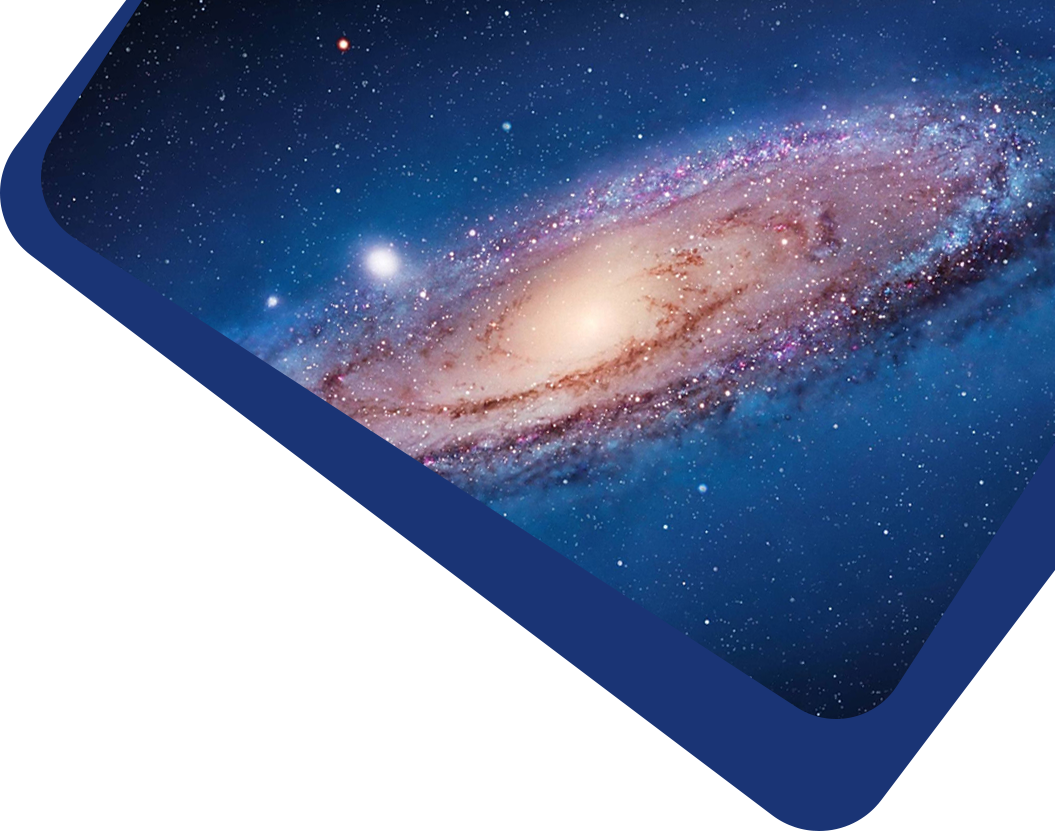Vol 18, No 3

## Statefinder diagnostic and constraints on the Palatini f(R) gravity theories

#### Shu-Lei Cao, Song Li, Hao-Ran Yu, Tong-Jie Zhang

Abstract

Abstract We focus on a series of f(R) gravity theories in Palatini formalism to investigate the probabilities of producing late-time acceleration for the flat Friedmann-Robertson-Walker (FRW) universe. We apply a statefinder diagnostic to these cosmological models for chosen series of parameters to see if they can be distinguished from one another. The diagnostic involves the statefinder pair {r, s}, where r is derived from the scale factor a and its higher derivatives with respect to the cosmic time t, and s is expressed by r and the deceleration parameter q. In conclusion, we find that although two types of f(R) theories: (i) f(R) = R + αRm − βR−n and (ii) f(R) = R + αln R − β can lead to late-time acceleration, their evolutionary trajectories in the r − s and r − q planes reveal different evolutionary properties, which certainly justify the merits of the statefinder diagnostic. Additionally, we utilize the observational Hubble parameter data (OHD) to constrain these models of f(R) gravity. As a result, except for m = n = 1/2 in case (i), α = 0 in case (i) and case (ii) allow the ΛCDM model to exist in the 1σ confidence region. After applying the statefinder diagnostic to the best-fit models, we find that all the best-fit models are capable of going through the deceleration/acceleration transition stage with a late-time acceleration epoch, and all these models turn to the de Sitter point ({r, s} = {1, 0}) in the future. Also, the evolutionary differences between these models are distinct, especially in the r − s plane, which makes the statefinder diagnostic more reliable in discriminating cosmological models.

Keywords

Keywords dark energy — cosmology: miscellaneous — methods: data analysis

Full Text
Refbacks

• There are currently no refbacks.